﻿ Spectrophotometric Investigation of Complexation of Ferrous Ion with 3,5-Dintrosalicylic AcidPublications are Open
Access in this journal
Article Versions
Export Article
• Normal Style
• MLA Style
• APA Style
• Chicago Style
Research Article
Open Access Peer-reviewed

### Spectrophotometric Investigation of Complexation of Ferrous Ion with 3,5-Dintrosalicylic Acid

Gurvinder Kaur, Sumanjeet Kaur, Tribhuvan Kumar Pathak, Vandana Sharma , Kartikay Tyagi
World Journal of Chemical Education. 2021, 9(3), 77-80. DOI: 10.12691/wjce-9-3-2
Received July 23, 2021; Revised August 29, 2021; Accepted September 07, 2021

### Abstract

The determination of stoichiometry and stability constant of metal-ligand complex holds great significance for chemical, physical, environmental and biological studies. In the present work, the stoichiometric ratio and stability constant of iron complex of 3,5-dinitrosalicylic acid was determined using Job’s method of continuous variation. Job’s method can be easily applied to investigate a wide range of colored complexes including mononuclear as well as polynuclear complexes of various species, therefore showing its usefulness for practical application in undergraduate University curriculum. The stoichiometry of the major complex formed was determined to have a 1:1 ratio of Fe+2 and 3,5-dinitrosalicylic acid. Stability constant value was determined and found to be 3 x 105. The resulting high stability of the complex can be attributed to resonance stabilization of metal-ligand П-bonding electron density. From the thermodynamic parameter, the negative value of overall change in Gibb’s free energy shows the complex formation between Fe+2 and 3,5-dinitrosalicylic acid is spontaneous and feasible.

### 1. Introduction

The determination of the stability constants and stoichiometries of the complexes is an essential subject in analytical chemistry. The stability constant and stoichiometry of a complex are frequently determined using a wide selection of traditional methods. These include: Bent and French 1, Harvey and Manning 2, Rose and Drago 3, Yoe and Jones 4, Job 5, Holme and Langmyhr 6, and Diehl and Lidstrom 7 methods. Out of these Job’s and Yoe and Jones’ methods are the most widely used due to the straightforward experimental application and simple theoretical foundation. These methods are based on the graphical representation of analytical signals versus molar fraction of ligand (Job’s method) or molar ratio of ligand to metal (Yoe and Jones’ method). In the representation, the analytical signal rises with the ligand molar ratio until a maximum is obtained; at this point, the signal falls (Job’s method) or it is maintained constant (Yoe and Jones’ method). The maxima of the obtained curve attributes to the maximum complex formation. This maxima indicates the major ligand fraction, which is the stoichiometry of the complex. In a similar way, the stability constant of the complex can be determined from the vertical distance that separates the maxima of the experimental curve and the intersection point of the tangents plotted on the curve.

However, despite the number of research work carried out for stability constant, it is still desirable to perform more of such determinations because of the numerous roles played by chelating ligands and their complexes in physical, chemical and bio-chemical environment. Therefore, it is necessary for undergraduate students of chemistry, bio-chemistry and biology to understand the importance of stoichiometry and stability constant of metal-ligand complex. Based on the available literature no studies have been reported for the determination of molar ratio and stability constant using spectrophotometer for bivalent transition metal ion iron(II) complex of 3,5-dinitrosalicylic acid (3,5-DNS). 3,5-DNS is a bidentate ligand which provides two coordination sites i.e., hydroxyl (-OH) and carboxyl (-COOH) groups. It is an important group of organic compounds used for determination of reducing sugars 8. The complexation tendency of this ligand has not yet been explored. Literature survey revealed that authors had reported the complex formation of 3,5-DNS with Pb+2 9, Cu+2 9, Mg+2 10, Be+2 11, Ni+2 12, Co+2 12, Zn+2 13, Mn+2 13, Hg+2 13 and Cd+2 13. It was considered worthwhile to extend the research on the interaction of bivalent transition metal ion Fe+2 with 3,5-DNS. Therefore in this paper, the stoichiometric ratio and stability constant of the Fe+2 complex of 3,5-DNS was determined using Job’s continuous variation method.

### 2. Theoretical Aspect

The formation of mononuclear complex can be represented by the following chemical equilibrium.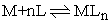Where M represents the metal ion, L is the ligand and n is the stoichiometric coefficient. On the basis of this chemical reaction, solutions of metal (CM) and ligand (CL) are prepared. The stoichiometry and stability of complex formed (MLn) is measured in accordance with the Job’s continuous variation method.

According to the Job’s method, the maximum amount of complex is formed at equilibrium when equimolar solutions of two reactants are mixed in varying proportions. When the reactants are colorless and complex is colored, the equilibrium concentration of the complex can be obtained by measuring absorbance (A) of the solution. The absorbance values are plotted versus the corresponding ligand molar fraction (χ), where χ = CL/C and C = CM + CL is a constant. A curve with a maxima provides corresponding a certain value of ligand molar fraction (χ) from which the stoichiometry can be deduced.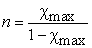(1)

The stability constant βn is given by expression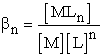(2)

Where [M], [L] and [MLn] are the equilibrium concentration of metal, ligand and complex respectively. Each one of these concentrations can be expressed in terms of the initial concentrations of ligand (CL) and (CM):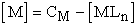(3)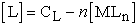(4)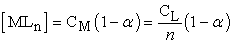(5)

Where α is the degree of dissociation of the complex. The concentrations terms CL and CM can also be expressed as: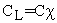(6)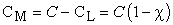(7)

The stability constant βn can be determined from the plots of the continuous variation method, where at the point of stoichiometry, the ratio between the observed absorbance (Aob) to the extrapolated absorbance (Aex) equal to the mole fraction of the metal ion or the ligand in the complex.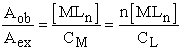(8)

Therefore, [MLn] can be expressed as: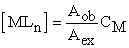(9)

Substituting [MLn] values in Eq. 3 & 4, the following equations are obtained.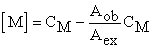(10)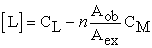(11)

Substituting Eq. 9, 10 & 11 in Eq. 2, the stability constant can be calculated from Eq.12 14.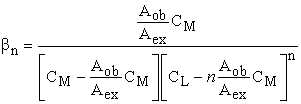(12)

### 3. Experimental Work

All the reagents used in the present work were of analytical grade (AR) and were used as received. Mohr’s salt, sodium potassium tartrate, acetic acid, 3,5-dinitrosalicylic acid (3,5-DNS) and hydroxyl amine hydrochloride were purchased from Sigma Aldrich. Sulphuric acid was obtained from Thomas Baker. Sodium hydroxide purchased from Sisco Research Laboratories Pvt. Ltd. Sodium acetate purchased from Qualigens. Distilled water was used throughout the experimental work.

All the weighing was carried out using Sartorious Quintix analytical balance. Spectrophotometric measurements were obtained from Systronics spectrophotometer. 0.001 M Mohr’s salt solution was prepared by dissolving 0.098g in 1 M H2SO4 solution in a 250 mL volumetric flask. 0.001 M 3,5- dinitrosalicylic acid (DNS) was prepared by dissolving 0.057 g of 3,5-DNS in 20 mL of 2N NaOH. Finally 3 g of sodium potassium tartrate was added and the remaining volume was filled with distilled water to make 250 mL of 3,5-DNS reagent.

Total nine stock solutions were prepared each of 25 mL, having volume of prepared Mohr’s salt and ligands solutions in varying proportions as given in the Table 1. Series of volumes (1, 2, 3, 4, 5, 6, 7, 8, 9 mL) of the 0.001 M Mohr’s salt solution were respectively pipetted into nine 25 mL volumetric flasks and aliquot (9, 8, 7, 6, 5, 4, 3, 2, 1 mL) of the 0.001 M ligand were added, keeping the total metal and ligand concentration constant.

To prevent the precipitation of iron salt, stock solutions were buffered at 4.5 pH using 5mL acetate buffer (75 mL of 0.1 M acetic acid + 25 mL of 0.1 M sodium acetate). Iron in ferrous state (Fe+2) can be readily air oxidized to ferric state (Fe+3) whose colored complex’s absorbance appeared at different wavelength than ferrous-ligand complex. Thus, we could not determine the total iron present by making measurement at only one wavelength. Therefore, 5 mL hydroxylamine hydrochloride (10 g in 100 mL distilled water) was added as a mild reducing agent before the color is developed. Finally the remaining volume was filled with distilled water to make 25 mL stock solution.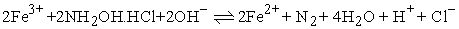The most colored stock solution was run in the UV-Vis spectrophotometer and varying the wavelengths from 400 to 800 nm to detect the maximum absorbance value corresponding it λmax. Then all other samples were run for their absorbance value at the selected λmax. To calculate the stoichiometry and stability constant, graph was plotted between absorbance and mole fraction of ligand in accordance with Job’s continuous variation method as described by Juan 15.

### 4. Results and Discussion

In Job’s method of continuous variation, solutions having various mole fractions were prepared for Fe+2 complex of 3,5-DNS. A portion from each solution was placed in the spectrophotometric cell and recorded their absorbance value at λmax=450 nm. Images of the prepared solutions are shown in Figure 1.

• Figure 1. Compositions of metal-ligand solutions with varying molar fractions

The compositions of each solution and their absorbance values are presented in Table 1. The ligand molar fraction was plotted against the absorbance as shown in Figure 2. Job’s plot exhibited maxima at 0.49 molar fraction (χmax) for Fe+2 complex of 3,5-DNS, from which coordination number (n) can be calculated using Eq. (1). The coordination number was found to be one (n=1), showing that iron reacts with 3,5-DNS in a molar ratio of 1:1. This value suggests that the 3,5-DNS bounds to the Fe+2 ion via two binding sites i.e., phenolic (-OH) and carboxylic (-COOH), resulting a 6 member stable chelate as shown in Figure 3.

Further, the stability constant of Fe+2 complex of 3,5-DNS was determined from the Job’s plot using Eq. (12). The resulting stability constant was 3 x 105, indicating high stability of the complex. The high stability can be attributed to resonance stabilization from benzene ring. With presence of two strong electrons withdrawing nitro groups in benzene ring, further stabilize the П-electrons density of metal-ligand П-bonding, thereby providing extra stability for the chelate. Also, chelate ring prevents complex from distortion either by compression or elongation of bonds, which consequently stabilizes the complex.

• Figure 2. Job’s plot for a 1:1 stoichiometry Fe+2 complex of 3,5-DNS
• Figure 3. Six member chelate formation between Fe+2 and 3,5-DNS

The value of overall changes in Gibb’s free energy (ΔG) for the formation of complex between Fe+2 and 3,5-DNS was evaluated at 25°C from the expression ΔG = -RTlnβn, where R is the universal gas constant and T is the absolute temperature. Gibb’s free energy change value was found to be -31.30 kJmol-1 suggesting a feasible complex formation occurred between Fe+2 ion and 3,5-DNS ligand.

### 5. Conclusion

The stoichiometry and stability constant of the metal complexes are important analytical and physical parameters. In the present work, we have determined the stoichiometry and stability constant of the Fe+2 complex of 3,5-DNS using Job’s continuous variation method. It was observed that there was a 1:1 ratio of Fe+2 to 3,5-DNS in the predominating complex because from Job’s plot, the ligand molar fraction at point maximum complex formation was 0.49. The expected value was 0.5. This discrepancy may be caused by propagated error due to equipment and apparatus used. The stability constant for the complexation was calculated to be 3 x 105. The very high value of stability constant and negative value of overall change in Gibb’s free energy further confirm the feasibility of the formation of iron and 3,5-DNS complex.

Job’s continuous variation method was used to determine stoichiometry, stability and thermodynamic parameter, which has an easier experimental procedure, can be easily applied to investigate numerous colored complexes including mononuclear as well as polynuclear complexes of various species. Therefore, this method is accepted for practical application in undergraduate University curriculum. A different method may be pursued for further confirmation, for example, using mass spectrometry or NMR to determine the stoichiometry and using potentiometric measurements to calculate stability constant.

### Acknowledgements

The authors are thankful to the Principal, SGTB Khalsa College, University of Delhi, Delhi, India, for the permission and continuous encouragement to pursue the present study. Authors are also thankful to laboratory staff of Department of Chemistry, SGTB Khalsa College, University of Delhi, for their consistent help throughout the experimental work.

### References

  Bent, H.E., French, C.L, “The structure of ferric thiocyanate and its dissociation constant in aqueous solution”,J. Am. Chem. Soc., 1941, 63(2), 568-572. In article View Article  Harvey, A.E., Manning, D.L, “Spectrophotometric methods of establishing empirical formulas of colored complexes in solution”, J. Am. Chem. Soc., 1950, 72(10), 4488-4493. In article View Article  Rose, N.J., Drago, R.S. “Molecular addition compounds of iodine. An absolute method for the spectroscopic determination of equilibrium constants”, J. Am. Chem. Soc., 1959, 81(23), 6138-6141. In article View Article  Yoe, J.H., Jones, A. L., Colorimetric determination of iron with disodium-1,2-dihydroxybenzene-3,5-disulfonate”, Ind. Eng. Chem. Anal. Ed., 1944, 16(2), 111-115. In article View Article  Job, P, “Formation and stability of inorganic complexes in solution”, Ann. Chim., 1928, 9, 113-203. In article  Holme, A., Langmyhr, F.R, “A modified and a new straight-line method for determining the composition of weak complexes of the form AmBn”, Anal. Chim. Acta., 1966, 36, 383-391. In article View Article  Diehl, H., Lindstrom, F, Anal. Chem., “Eriochrome Black T and its calcium and magnesium derivatives” Anal. Chem. 1959,31(3), 414-418. In article View Article  Miller, G.L, “Use of dinitrosalicylic acid reagent for determination of reducing sugar” Anal. Chem., 1959, 31(3), 426-428. In article View Article  Dube, S.S., Dhindsa, S.S, J. Ind. Chem. Soc., 1969, 46, 838. In article  Dube, S.S., Dhindsa, S.S, “Potentiometric study on the composition and stability of beryllium complexes of 3,5-dinitrosalicylic acid” Z. Naturforsch, 1969, 24(8), 967-970. In article View Article  Dube, S.S., Dhindsa, S.S, Naturforsch, “Potentiometric investigation on the 3,5-dinitrosalicyclic acid complex of Mg(II).” 1969, 24(10), 1234-1236. In article View Article  Dube, S.S., Dhindsa, S.S, Indian, J. Chem., 1969, 7, 823. In article  Dube, S.S., Dhindsa, S.S, “Composition and stability of some bivalent transistion metal complexes of 3,5-dinitrosalicylic acid” Canad. J. Chem., 1970, 48, 1007-1010. In article View Article  Inczedy, J. “Analytical applications of complex equilibria”. Ellis horwood series in analytical Chemistry. Wiley, New York, 1976. In article  Bosque-Sendra, J.M., almansa-Lopez, E., Garcia-Campana, A.M., Cuadros-Rodriguez, L, “Data Analysis in the determination of stoichiometries and stability constants of complexes” Anal. Sci., 2003, 19, 1431-1439. In article View Article  PubMed

Published with license by Science and Education Publishing, Copyright © 2021 Gurvinder Kaur, Sumanjeet Kaur, Tribhuvan Kumar Pathak, Vandana Sharma and Kartikay TyagiThis work is licensed under a Creative Commons Attribution 4.0 International License. To view a copy of this license, visit http://creativecommons.org/licenses/by/4.0/